# polyfit

Polynomial curve fitting

## Syntax

``p = polyfit(x,y,n)``
``````[p,S] = polyfit(x,y,n)``````
``````[p,S,mu] = polyfit(x,y,n)``````

## Description

example

````p = polyfit(x,y,n)` returns the coefficients for a polynomial `p(x)` of degree `n` that is a best fit (in a least-squares sense) for the data in `y`. The coefficients in `p` are in descending powers, and the length of `p` is `n+1`$p\left(x\right)={p}_{1}{x}^{n}+{p}_{2}{x}^{n-1}+...+{p}_{n}x+{p}_{n+1}.$```
``````[p,S] = polyfit(x,y,n)``` also returns a structure `S` that can be used as an input to `polyval` to obtain error estimates.```

example

``````[p,S,mu] = polyfit(x,y,n)``` performs centering and scaling to improve the numerical properties of both the polynomial and the fitting algorithm. This syntax additionally returns `mu`, which is a two-element vector with centering and scaling values. `mu(1)` is `mean(x)`, and `mu(2)` is `std(x)`. Using these values, `polyfit` centers `x` at zero and scales it to have unit standard deviation,$\stackrel{^}{x}=\frac{x-\overline{x}}{{\sigma }_{x}}\text{\hspace{0.17em}}.$```

## Examples

collapse all

Generate 10 points equally spaced along a sine curve in the interval `[0,4*pi]`.

```x = linspace(0,4*pi,10); y = sin(x);```

Use `polyfit` to fit a 7th-degree polynomial to the points.

`p = polyfit(x,y,7);`

Evaluate the polynomial on a finer grid and plot the results.

```x1 = linspace(0,4*pi); y1 = polyval(p,x1); figure plot(x,y,'o') hold on plot(x1,y1) hold off```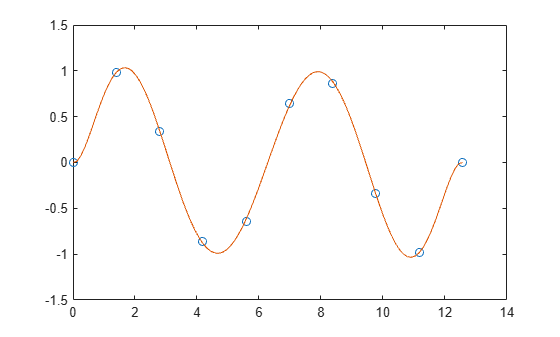Create a vector of 5 equally spaced points in the interval `[0,1]`, and evaluate $y\left(x\right)=\left(1+x{\right)}^{-1}$ at those points.

```x = linspace(0,1,5); y = 1./(1+x);```

Fit a polynomial of degree 4 to the 5 points. In general, for `n` points, you can fit a polynomial of degree `n-1` to exactly pass through the points.

`p = polyfit(x,y,4);`

Evaluate the original function and the polynomial fit on a finer grid of points between 0 and 2.

```x1 = linspace(0,2); y1 = 1./(1+x1); f1 = polyval(p,x1);```

Plot the function values and the polynomial fit in the wider interval `[0,2]`, with the points used to obtain the polynomial fit highlighted as circles. The polynomial fit is good in the original `[0,1]` interval, but quickly diverges from the fitted function outside of that interval.

```figure plot(x,y,'o') hold on plot(x1,y1) plot(x1,f1,'r--') legend('y','y1','f1')```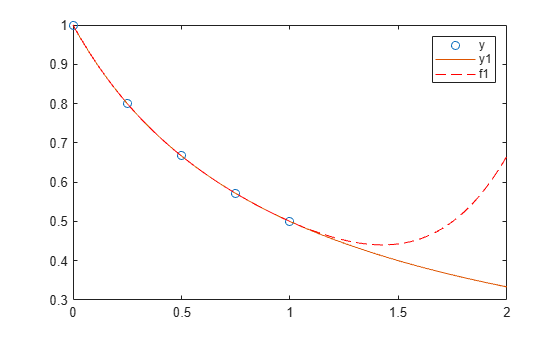First generate a vector of `x` points, equally spaced in the interval `[0,2.5]`, and then evaluate `erf(x)` at those points.

```x = (0:0.1:2.5)'; y = erf(x);```

Determine the coefficients of the approximating polynomial of degree 6.

`p = polyfit(x,y,6)`
```p = 1×7 0.0084 -0.0983 0.4217 -0.7435 0.1471 1.1064 0.0004 ```

To see how good the fit is, evaluate the polynomial at the data points and generate a table showing the data, fit, and error.

```f = polyval(p,x); T = table(x,y,f,y-f,'VariableNames',{'X','Y','Fit','FitError'})```
```T=26×4 table X Y Fit FitError ___ _______ __________ ___________ 0 0 0.00044117 -0.00044117 0.1 0.11246 0.11185 0.00060836 0.2 0.2227 0.22231 0.00039189 0.3 0.32863 0.32872 -9.7429e-05 0.4 0.42839 0.4288 -0.00040661 0.5 0.5205 0.52093 -0.00042568 0.6 0.60386 0.60408 -0.00022824 0.7 0.6778 0.67775 4.6383e-05 0.8 0.7421 0.74183 0.00026992 0.9 0.79691 0.79654 0.00036515 1 0.8427 0.84238 0.0003164 1.1 0.88021 0.88005 0.00015948 1.2 0.91031 0.91035 -3.9919e-05 1.3 0.93401 0.93422 -0.000211 1.4 0.95229 0.95258 -0.00029933 1.5 0.96611 0.96639 -0.00028097 ⋮ ```

In this interval, the interpolated values and the actual values agree fairly closely. Create a plot to show how outside this interval, the extrapolated values quickly diverge from the actual data.

```x1 = (0:0.1:5)'; y1 = erf(x1); f1 = polyval(p,x1); figure plot(x,y,'o') hold on plot(x1,y1,'-') plot(x1,f1,'r--') axis([0 5 0 2]) hold off```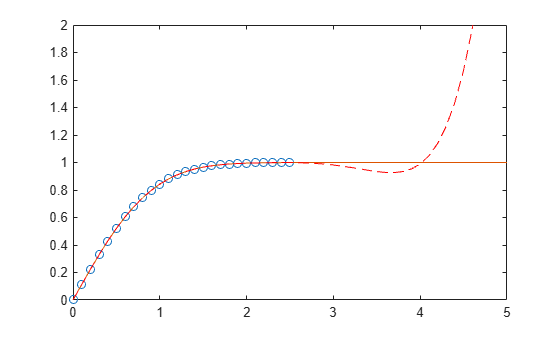Create a table of population data for the years 1750 - 2000 and plot the data points.

```year = (1750:25:2000)'; pop = 1e6*[791 856 978 1050 1262 1544 1650 2532 6122 8170 11560]'; T = table(year, pop)```
```T=11×2 table year pop ____ _________ 1750 7.91e+08 1775 8.56e+08 1800 9.78e+08 1825 1.05e+09 1850 1.262e+09 1875 1.544e+09 1900 1.65e+09 1925 2.532e+09 1950 6.122e+09 1975 8.17e+09 2000 1.156e+10 ```
`plot(year,pop,'o')`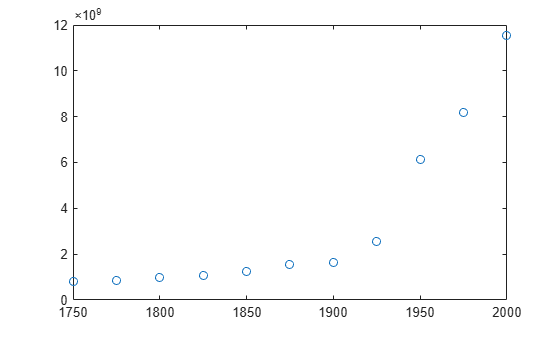Use `polyfit` with three outputs to fit a 5th-degree polynomial using centering and scaling, which improves the numerical properties of the problem. `polyfit` centers the data in `year` at 0 and scales it to have a standard deviation of 1, which avoids an ill-conditioned Vandermonde matrix in the fit calculation.

`[p,~,mu] = polyfit(T.year, T.pop, 5);`

Use `polyval` with four inputs to evaluate `p` with the scaled years, `(year-mu(1))/mu(2)`. Plot the results against the original years.

```f = polyval(p,year,[],mu); hold on plot(year,f) hold off```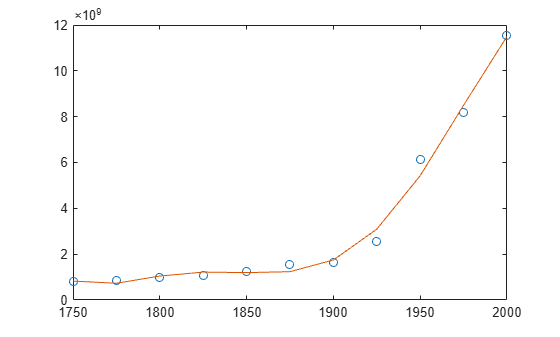Fit a simple linear regression model to a set of discrete 2-D data points.

Create a few vectors of sample data points (x,y). Fit a first degree polynomial to the data.

```x = 1:50; y = -0.3*x + 2*randn(1,50); p = polyfit(x,y,1); ```

Evaluate the fitted polynomial `p` at the points in `x`. Plot the resulting linear regression model with the data.

```f = polyval(p,x); plot(x,y,'o',x,f,'-') legend('data','linear fit') ```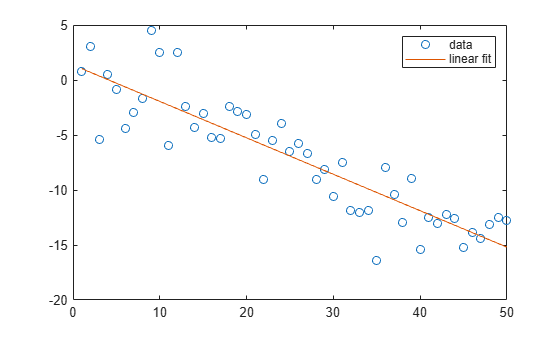Fit a linear model to a set of data points and plot the results, including an estimate of a 95% prediction interval.

Create a few vectors of sample data points (x,y). Use `polyfit` to fit a first degree polynomial to the data. Specify two outputs to return the coefficients for the linear fit as well as the error estimation structure.

```x = 1:100; y = -0.3*x + 2*randn(1,100); [p,S] = polyfit(x,y,1); ```

Evaluate the first-degree polynomial fit in `p` at the points in `x`. Specify the error estimation structure as the third input so that `polyval` calculates an estimate of the standard error. The standard error estimate is returned in `delta`.

`[y_fit,delta] = polyval(p,x,S);`

Plot the original data, linear fit, and 95% prediction interval $\mathit{y}±2\Delta$.

```plot(x,y,'bo') hold on plot(x,y_fit,'r-') plot(x,y_fit+2*delta,'m--',x,y_fit-2*delta,'m--') title('Linear Fit of Data with 95% Prediction Interval') legend('Data','Linear Fit','95% Prediction Interval')```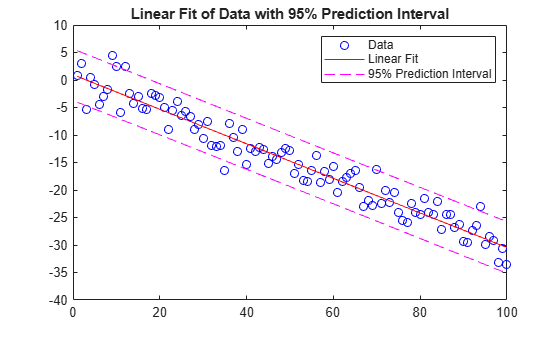## Input Arguments

collapse all

Query points, specified as a vector. The points in `x` correspond to the fitted function values contained in `y`. If `x` is not a vector, then `polyfit` converts it into a column vector `x(:)`.

Warning messages result when `x` has repeated (or nearly repeated) points or if `x` might need centering and scaling.

Data Types: `single` | `double`
Complex Number Support: Yes

Fitted values at query points, specified as a vector. The values in `y` correspond to the query points contained in `x`. If `y` is not a vector, then `polyfit` converts it into a column vector `y(:)`.

Data Types: `single` | `double`
Complex Number Support: Yes

Degree of polynomial fit, specified as a positive integer scalar. `n` specifies the polynomial power of the left-most coefficient in `p`.

## Output Arguments

collapse all

Least-squares fit polynomial coefficients, returned as a vector. `p` has length `n+1` and contains the polynomial coefficients in descending powers, with the highest power being `n`. If either `x` or `y` contain `NaN` values and `n < length(x)`, then all elements in `p` are `NaN`. If you specify three output arguments to center and scale the data, then `polyfit` returns different coefficients in `p` compared to when the data is not centered and scaled.

Use `polyval` to evaluate `p` at query points.

Error estimation structure. This optional output structure is primarily used as an input to the `polyval` function to obtain error estimates. `S` contains the following fields:

FieldDescription
`R`Triangular `R` factor (possibly permuted) from a QR decomposition of the Vandermonde matrix of `x`
`df`Degrees of freedom
`normr`Norm of the residuals

If the data in `y` is random, then an estimate of the covariance matrix of `p` is `(Rinv*Rinv')*normr^2/df`, where `Rinv` is the inverse of `R`.

If the errors in the data in `y` are independent and normal with constant variance, then `[y,delta] = polyval(...)` produces error bounds that contain at least 50% of the predictions. That is, `y` ± `delta` contains at least 50% of the predictions of future observations at `x`.

Centering and scaling values, returned as a two-element vector. `mu(1)` is `mean(x)`, and `mu(2)` is `std(x)`. These values center the query points in `x` at zero with unit standard deviation.

Use `mu` as the fourth input to `polyval` to evaluate `p` at the scaled points, ```(x - mu(1))/mu(2)```.

## Limitations

• In problems with many points, increasing the degree of the polynomial fit using `polyfit` does not always result in a better fit. High-order polynomials can be oscillatory between the data points, leading to a poorer fit to the data. In those cases, you might use a low-order polynomial fit (which tends to be smoother between points) or a different technique, depending on the problem.

• Polynomials are unbounded, oscillatory functions by nature. Therefore, they are not well-suited to extrapolating bounded data or monotonic (increasing or decreasing) data.

## Algorithms

`polyfit` uses `x` to form Vandermonde matrix `V` with `n+1` columns and `m = length(x)` rows, resulting in the linear system

`$\left(\begin{array}{cccc}{x}_{1}^{n}& {x}_{1}^{n-1}& \cdots & 1\\ {x}_{2}^{n}& {x}_{2}^{n-1}& \cdots & 1\\ ⋮& ⋮& \ddots & ⋮\\ {x}_{m}^{n}& {x}_{m}^{n-1}& \cdots & 1\end{array}\right)\left(\begin{array}{c}{p}_{1}\\ {p}_{2}\\ ⋮\\ {p}_{n+1}\end{array}\right)=\left(\begin{array}{c}{y}_{1}\\ {y}_{2}\\ ⋮\\ {y}_{m}\end{array}\right)\text{\hspace{0.17em}}\text{\hspace{0.17em}},$`

which `polyfit` solves with `p = V\y`. Since the columns in the Vandermonde matrix are powers of the vector `x`, the condition number of `V` is often large for high-order fits, resulting in a singular coefficient matrix. In those cases centering and scaling can improve the numerical properties of the system to produce a more reliable fit.

## Version History

Introduced before R2006a